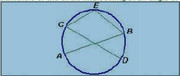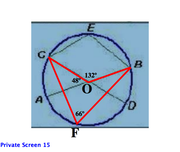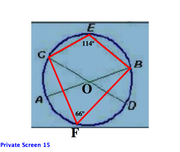GMAT Question of the Day: Daily via email | Daily via Instagram New to GMAT Club? Watch this Video

 It is currently 23 Jan 2020, 04:47### GMAT Club Daily Prep

#### Thank you for using the timer - this advanced tool can estimate your performance and suggest more practice questions. We have subscribed you to Daily Prep Questions via email.

Customized
for You

we will pick new questions that match your level based on your Timer History

Track

every week, we’ll send you an estimated GMAT score based on your performance

Practice
Pays

we will pick new questions that match your level based on your Timer History

#### Not interested in getting valuable practice questions and articles delivered to your email? No problem, unsubscribe here.# In the figure AB and CD are two diameters of circle. Interse

Author Message
TAGS:

### Hide Tags

ManagerB
Joined: 10 Mar 2014
Posts: 177
In the figure AB and CD are two diameters of circle. Interse  [#permalink]

### Show Tags

8
5600:00

Difficulty:75% (hard)

Question Stats:58% (02:36) correct42% (02:40) wrongbased on 461 sessions

### HideShow timer Statistics

Attachment:Circle_Angle.JPG [ 16.89 KiB | Viewed 11452 times ]
In the figure AB and CD are two diameters of circle. Intersecting at angle 48 degree. E is any point on Arc CB. find angle CEB

A. 114
B. 100
C. 80
D. 96
E. 40

Originally posted by PathFinder007 on 03 Aug 2014, 02:28.
Last edited by Bunuel on 04 Dec 2014, 04:32, edited 1 time in total.
Edited the question.
TutorJoined: 20 Apr 2012
Posts: 96
Location: Ukraine
GMAT 1: 690 Q51 V31
GMAT 2: 730 Q51 V38
WE: Education (Education)
Re: In the figure AB and CD are two diameters of circle. Interse  [#permalink]

### Show Tags

12
7
desaichinmay22 wrote:
smyarga wrote:
Since, CD and AB are two diameters of the circle, so their intersection is the centre. Let's denote this centre O. Angle CEB is inscribed, and angle COB is corresponding central angle (arc CAB). The value of angle COB=180+48=228 degrees. The measure of inscribed angle is always half the measure of the central angle. Angle CEB is equal =228/2=114 degrees.

Hi,

I thought angle COB is 180-42=138 degrees. You have derived angle COB of 228 degrees.

That's why I mentioned arc CAB. You don't really need to understand the notions of major and minor arc. Corresponding central angle means that it stays on the same arc as your inscribed angle.
Attachmentscircle.png [ 41.27 KiB | Viewed 11210 times ]

##### General Discussion
TutorJoined: 20 Apr 2012
Posts: 96
Location: Ukraine
GMAT 1: 690 Q51 V31
GMAT 2: 730 Q51 V38
WE: Education (Education)
Re: In the figure AB and CD are two diameters of circle. Interse  [#permalink]

### Show Tags

13
4
Since, CD and AB are two diameters of the circle, so their intersection is the centre. Let's denote this centre O. Angle CEB is inscribed, and angle COB is corresponding central angle (arc CAB). The value of angle COB=180+48=228 degrees. The measure of inscribed angle is always half the measure of the central angle. Angle CEB is equal =228/2=114 degrees.

InternJoined: 01 Apr 2013
Posts: 16
Schools: Tepper '16 (S)
Re: In the figure AB and CD are two diameters of circle. Interse  [#permalink]

### Show Tags

5
desaichinmay22 wrote:
smyarga wrote:
Since, CD and AB are two diameters of the circle, so their intersection is the centre. Let's denote this centre O. Angle CEB is inscribed, and angle COB is corresponding central angle (arc CAB). The value of angle COB=180+48=228 degrees. The measure of inscribed angle is always half the measure of the central angle. Angle CEB is equal =228/2=114 degrees.

Hi,

I thought angle COB is 180-42=138 degrees. You have derived angle COB of 228 degrees.

Hi buddy,

COB is indeed 180-48 = 132 degrees . There is a concept of major and minor segment ( please google this ) Now the point O is in the minor segment of the arc and in this case it would be half of the angle subtended in the major segment i.e ( 360-132) = 228 /2 = 114 .
TutorJoined: 20 Apr 2012
Posts: 96
Location: Ukraine
GMAT 1: 690 Q51 V31
GMAT 2: 730 Q51 V38
WE: Education (Education)
Re: In the figure AB and CD are two diameters of circle. Interse  [#permalink]

### Show Tags

2
1
desaichinmay22, specially for you another nice problem with central angle if-the-radius-of-the-circle-below-see-attachment-is-equal-175709.html
Senior ManagerB
Joined: 14 Jul 2013
Posts: 275
Location: India
Concentration: Marketing, Strategy
GMAT 1: 690 Q49 V34
GMAT 2: 670 Q49 V33
GPA: 3.6
WE: Brand Management (Retail)
Re: In the figure AB and CD are two diameters of circle. Interse  [#permalink]

### Show Tags

2
PathFinder007 wrote:
In the figure AB and CD are two diameters of circle. Intersecting at angle 48 degree. E is any point on Arc CB. find angle CEB

A 114
B 100
C 80
D 96
E 40

Join Segment AE in the figure, we know Angle AEC is half of Angle COA(Assuming diameter intersect at point O).

Angle AEC = 24
Ab is diameter, so Angle AEB = 90
Angle CEB = 90+24 = 114
_________________
GMAT Club LegendV
Joined: 12 Sep 2015
Posts: 4224
Re: In the figure AB and CD are two diameters of circle. Interse  [#permalink]

### Show Tags

2
Top Contributor
PathFinder007 wrote:
Attachment:
Circle_Angle.JPG
In the figure AB and CD are two diameters of circle. Intersecting at angle 48 degree. E is any point on Arc CB. find angle CEB

A. 114
B. 100
C. 80
D. 96
E. 40

Let's use some useful circle properties

First let's add a blue line, to divide ∠CEB into 2 anglesCD is the DIAMETER of the circle
Since ∠CED is an inscribed angle containing (aka "holding") the diameter, we can conclude that ∠CED = 90°Now recognize that the intersection of AB and CD creates two equal (vertically opposite) angles, we can conclude the angle opposite the 48° is also 48°Now recognize that we have two angles containing (aka "holding") the arc BD
Property: If a CENTRAL angle and an INSCRIBED angle contain the same arc, then the CENTRAL angle is TWICE the INSCRIBED angle
This means that ∠DEB = 24°At this point, we have:So, ∠CEB = 90° + 24° = 114°

RELATED VIDEO FROM OUR COURSE

_________________
TutorJoined: 20 Apr 2012
Posts: 96
Location: Ukraine
GMAT 1: 690 Q51 V31
GMAT 2: 730 Q51 V38
WE: Education (Education)
Re: In the figure AB and CD are two diameters of circle. Interse  [#permalink]

### Show Tags

1
PathFinder007 wrote:
In the figure AB and CD are two diameters of circle. Intersecting at angle 48 degree. E is any point on Arc CB. find angle CEB

A 114
B 100
C 80
D 96
E 40

Kudos from me, because I love problems with central angles:)
ManagerJoined: 21 Sep 2012
Posts: 206
Location: United States
Concentration: Finance, Economics
Schools: CBS '17
GPA: 4
WE: General Management (Consumer Products)
Re: In the figure AB and CD are two diameters of circle. Interse  [#permalink]

### Show Tags

1
smyarga wrote:
Since, CD and AB are two diameters of the circle, so their intersection is the centre. Let's denote this centre O. Angle CEB is inscribed, and angle COB is corresponding central angle (arc CAB). The value of angle COB=180+48=228 degrees. The measure of inscribed angle is always half the measure of the central angle. Angle CEB is equal =228/2=114 degrees.

Hi,

I thought angle COB is 180-42=138 degrees. You have derived angle COB of 228 degrees.
ManagerB
Joined: 06 Aug 2013
Posts: 62
Re: In the figure AB and CD are two diameters of circle. Interse  [#permalink]

### Show Tags

1
smyarga wrote:
desaichinmay22 wrote:
smyarga wrote:
Since, CD and AB are two diameters of the circle, so their intersection is the centre. Let's denote this centre O. Angle CEB is inscribed, and angle COB is corresponding central angle (arc CAB). The value of angle COB=180+48=228 degrees. The measure of inscribed angle is always half the measure of the central angle. Angle CEB is equal =228/2=114 degrees.

Hi,

I thought angle COB is 180-42=138 degrees. You have derived angle COB of 228 degrees.

That's why I mentioned arc CAB. You don't really need to understand the notions of major and minor arc. Corresponding central angle means that it stays on the same arc as your inscribed angle.

another approach,

join C and A. since AB is the diameter, A, C, E and B form a cyclic quadrilateral. now opposit angles of cyclic quadrilaterals sum up to 180 degrees. considering the point of intersection of the 2 diameters as O.

angle AOC = 48 degrees. ........... given
OA = OC, radius of the circle, hence trianlge OAC is isoceles.
thus angle ACO = angle OAC = 66 degrees.

now,
angle OAC + angle CEB = 180 degrees......... since opposit angles of cyclic quadrilaterals sum up to 180 degrees.
therefore, angle CEB = 180 - 66 = 114 degrees.

kudos if you like.GMAT TutorG
Status: Tutor - BrushMyQuant
Joined: 05 Apr 2011
Posts: 635
Location: India
Concentration: Finance, Marketing
Schools: XLRI (A)
GMAT 1: 700 Q51 V31
GPA: 3
WE: Information Technology (Computer Software)
Re: In the figure AB and CD are two diameters of circle. Interse  [#permalink]

### Show Tags

1
Thank you!
_________________
DirectorG
Joined: 04 Aug 2010
Posts: 514
Schools: Dartmouth College
Re: In the figure AB and CD are two diameters of circle. Interse  [#permalink]

### Show Tags

1
1
Quote:In the figure AB and CD are two diameters of circle. Intersecting at angle 48 degree. E is any point on Arc CB. find angle CEB

A. 114
B. 100
C. 80
D. 96
E. 40

An INSCRIBED ANGLE is formed by two chords.
A CENTRAL ANGLE is formed by two radii.
When an inscribed angle and a central angle intercept the same two points on a circle, the inscribed angle is 1/2 the central angle.Here, inscribed angle CFB and central angle COB both intercept the circle at points C and B.
Since central angle COB = 132º, inscribed angle CFB = (1/2)(132) = 66º.

Rule:
When a quadrilateral is inscribed in a circle, opposite angles sum to 180º.Since opposite angles inside inscribed quadrilateral CEBF must sum to 180º, angle CEB = 180-66 = 114.

_________________
GMAT and GRE Tutor
New York, NY

Available for tutoring in NYC and long-distance.
Math ExpertV
Joined: 02 Aug 2009
Posts: 8335
Re: In the figure AB and CD are two diameters of circle. Interse  [#permalink]

### Show Tags

1
ArupRS wrote:

Dear experts,

I thought smaller angle COB is 48 degree. Hence the angle CEB is half of angle COB. Please explain what I am missing here.

Regards,
Arup

No COB is not 48..
Even if you take COB as 48, CEB is not half of COB..
Any point say r in major arc CB that is CADB will make an angle CrB which will be half of COB.
This becomes half of 48 so 24..
Now CEB+CrB will be 180 as sum of opposite angles of a cyclic quadrilateral is 180 and CEBr is cyclic quadrilateral.
Thus CEB = 180-24=156..
But no choice is given as 156.

So you have to take COB as 180-48=132
And CEB = 180-132/2=180-66=114
_________________
Veritas Prep GMAT InstructorV
Joined: 16 Oct 2010
Posts: 10004
Location: Pune, India
Re: In the figure AB and CD are two diameters of circle. Interse  [#permalink]

### Show Tags

1
ArupRS wrote:

Dear experts,

I thought smaller angle COB is 48 degree. Hence the angle CEB is half of angle COB. Please explain what I am missing here.

Regards,
Arup

Angle COB is the larger angle so it will not be 48 degrees.
Also, inscribed angle is half of the central angle that subtends the same arc.

COB and CEB subtend different arcs.
Angle COB subtends arc CEB.
So angle COB will be twice of angle CAB or CDB.
_________________
Karishma
Veritas Prep GMAT Instructor

InternJoined: 31 Jul 2014
Posts: 18
Re: In the figure AB and CD are two diameters of circle. Interse  [#permalink]

### Show Tags

desaichinmay22 wrote:
smyarga wrote:
Since, CD and AB are two diameters of the circle, so their intersection is the centre. Let's denote this centre O. Angle CEB is inscribed, and angle COB is corresponding central angle (arc CAB). The value of angle COB=180+48=228 degrees. The measure of inscribed angle is always half the measure of the central angle. Angle CEB is equal =228/2=114 degrees.

Hi,

I thought angle COB is 180-42=138 degrees. You have derived angle COB of 228 degrees.

According to me, CB is not the diameter. So central angle doesnt apply in this case. Also, COB is 138 and not 228.
ManagerJoined: 21 Sep 2012
Posts: 206
Location: United States
Concentration: Finance, Economics
Schools: CBS '17
GPA: 4
WE: General Management (Consumer Products)
Re: In the figure AB and CD are two diameters of circle. Interse  [#permalink]

### Show Tags

smyarga wrote:
desaichinmay22 wrote:
smyarga wrote:
Since, CD and AB are two diameters of the circle, so their intersection is the centre. Let's denote this centre O. Angle CEB is inscribed, and angle COB is corresponding central angle (arc CAB). The value of angle COB=180+48=228 degrees. The measure of inscribed angle is always half the measure of the central angle. Angle CEB is equal =228/2=114 degrees.

Hi,

I thought angle COB is 180-42=138 degrees. You have derived angle COB of 228 degrees.

That's why I mentioned arc CAB. You don't really need to understand the notions of major and minor arc. Corresponding central angle means that it stays on the same arc as your inscribed angle.

Now I got the explanation. Thanks for the help.
InternJoined: 15 Jul 2012
Posts: 32
Re: In the figure AB and CD are two diameters of circle. Interse  [#permalink]

### Show Tags

smyarga wrote:
desaichinmay22 wrote:
smyarga wrote:
Since, CD and AB are two diameters of the circle, so their intersection is the centre. Let's denote this centre O. Angle CEB is inscribed, and angle COB is corresponding central angle (arc CAB). The value of angle COB=180+48=228 degrees. The measure of inscribed angle is always half the measure of the central angle. Angle CEB is equal =228/2=114 degrees.

Hi,

I thought angle COB is 180-42=138 degrees. You have derived angle COB of 228 degrees.

That's why I mentioned arc CAB. You don't really need to understand the notions of major and minor arc. Corresponding central angle means that it stays on the same arc as your inscribed angle.

Hi Smyarga,
how did you get 180 degrees?
i thought about vertically opposite angles then realised that i was wrong.
TutorJoined: 20 Apr 2012
Posts: 96
Location: Ukraine
GMAT 1: 690 Q51 V31
GMAT 2: 730 Q51 V38
WE: Education (Education)
Re: In the figure AB and CD are two diameters of circle. Interse  [#permalink]

### Show Tags

saggii27 wrote:
smyarga wrote:
desaichinmay22 wrote:

Hi,

I thought angle COB is 180-42=138 degrees. You have derived angle COB of 228 degrees.

That's why I mentioned arc CAB. You don't really need to understand the notions of major and minor arc. Corresponding central angle means that it stays on the same arc as your inscribed angle.

Hi Smyarga,
how did you get 180 degrees?
i thought about vertically opposite angles then realised that i was wrong.

Since COD is straight.
ManagerJoined: 25 Apr 2014
Posts: 92
Re: In the figure AB and CD are two diameters of circle. Interse  [#permalink]

### Show Tags

Hi there,
Can someone explain me this with the help of proper diagram,unable to get it!
Math ExpertV
Joined: 02 Sep 2009
Posts: 60625
Re: In the figure AB and CD are two diameters of circle. Interse  [#permalink]

### Show Tags

nktdotgupta wrote:
Thank you!

Moved to PS forum. Thank you for noticing this.
_________________Re: In the figure AB and CD are two diameters of circle. Interse   [#permalink] 15 Dec 2014, 08:11

Go to page    1   2    Next  [ 36 posts ]

Display posts from previous: Sort by

# In the figure AB and CD are two diameters of circle. Interse